Search

Synthetic Division Examples (page 2 of 4)

Sections: Introduction, Worked examples, Finding zeroes, Factoring polynomials

• Complete the indicated division.
•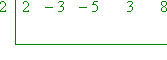For this first exercise, I will display the entire synthetic-division process step-by-step.

 First, carry down the "2" that indicates the leading coefficient: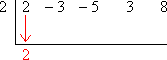Multiply by the number on the left, and carry the result into the next column: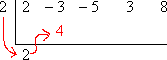Add down the column: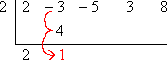Multiply by the number on the left, and carry the result into the next column: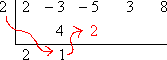Add down the column: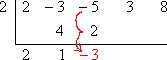Multiply by the number on the left, and carry the result into the next column: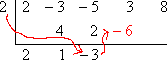Add down the column: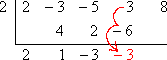Multiply by the number on the left, and carry the result into the next column: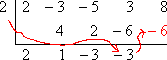Add down the column for the remainder: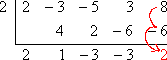The completed division is: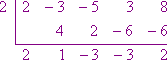This exercise never said anything about polynomials, factors, or zeroes, but this division says that, if you divide 2x4  3x3  5x2 + 3x + 8 by x  2, then the remainder will be 2, and therefore x  2 is not a factor of 2x4  3x3  5x2 + 3x + 8, and x = 2 is not a zero (that is, a root or x-intercept) of the initial polynomial. Copyright © Elizabeth Stapel 2002-2011 All Rights Reserved

• Divide 3x3  2x2 + 3x  4 by x  3 using synthetic division.
Write the answer in the form "
q(x) + r(x)/d(x) ".
• This question is asking me, in effect, to convert an "improper" polynomial "fraction" into a polynomial "mixed number". That is, I am being asked to do something similar to converting the improper fraction 17/5 to the mixed number 3 2/5, which is really the shorthand for the addition expression "3 + 2/5".

To convert the polynomial division into the required "mixed number" format, I have to do the division; I will show most of the steps.

 First, write down all the coefficients, and put the zero from x  3 = 0 (so x = 3) at the left.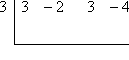Next, carry down the leading coefficient: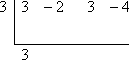Multiply by the potential zero, carry up to the next column, and add down: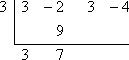Repeat this process:Repeat this process again: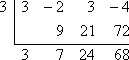As you can see, the remainder is 68. Since I started with a polynomial of degree 3 and then divided by x  3 (that is, by a polynomial of degree 1), I am left with a polynomial of degree 2. Then the bottom line represents the polynomial 3x2 + 7x + 24 with a remainder of 68. Putting this result into the required "mixed number" format, I get the answer as being: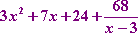It is always true that, when you use synthetic division, your answer (in the bottom row) will be of degree one less than what you'd started with, because you have divided out a linear factor. That was how I knew that my answer, denoted by the "3  7  24" in the bottom row, stood for a quadratic, since I had started with a cubic.

<< Previous  Top  |  1 | 2 | 3 | 4  |  Return to Index  Next >>

 Cite this article as: Stapel, Elizabeth. "Synthetic Division Examples." Purplemath. Available from     https://www.purplemath.com/modules/synthdiv2.htm. Accessed [Date] [Month] 2016

Study Skills Survey

Tutoring from Purplemath
Find a local math tutor

 Copyright © 2021  Elizabeth Stapel   |   About   |   Terms of Use   |   Linking   |   Site Licensing Contact Us